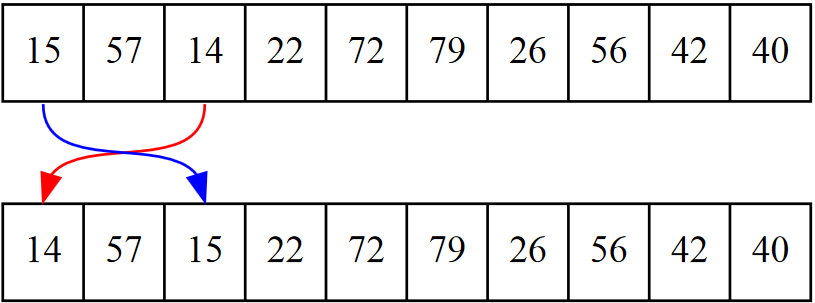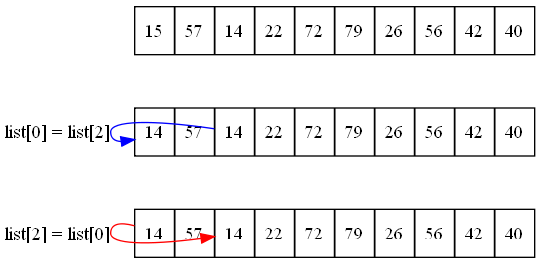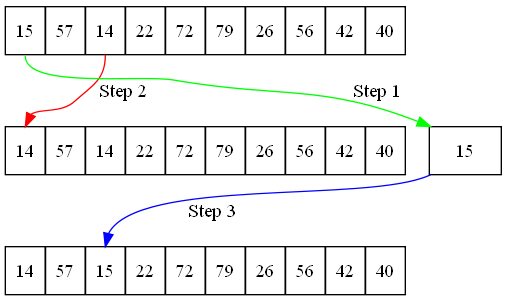﻿ Program Arcade Games With Python And Pygame

Program Arcade GamesWith Python And Pygame

 < Previous Home Next >

Chapter 17: Sorting

Binary searches only work on lists that are in order. So how do programs get a list in order? How does a program sort a list of items when the user clicks a column heading, or otherwise needs something sorted?

There are several algorithms that do this. The two easiest algorithms for sorting are the selection sort and the insertion sort. Other sorting algorithms exist as well, such as the shell, merge, heap, and quick sorts.

The best way to get an idea on how these sorts work is to watch them. To see common sorting algorithms in action visit this excellent website:
http://www.sorting-algorithms.com

Each sort has advantages and disadvantages. Some sort a list quickly if the list is almost in order to begin with. Some sort a list quickly if the list is in a completely random order. Other lists sort fast, but take more memory. Understanding how sorts work is important in selecting the proper sort for your program.

17.1 Swapping Values

Before learning to sort, we need to learn how to swap values between two variables. This is a common operation in many sorting algorithms. Suppose a program has a list that looks like the following:

my_list = [15,57,14,33,72,79,26,56,42,40]

The developer wants to swap positions 0 and 2, which contain the numbers 15 and 14 respectively. See Figure 17.1.Figure 17.1: Swapping values in an array

A first attempt at writing this code might look something like this:

my_list = my_list
my_list = my_listFigure 17.2: Incorrect attempt to swap array values

See Figure 17.2 to get an idea on what would happen. This clearly does not work. The first assignment list = list causes the value 15 that exists in position 0 to be overwritten with the 14 in position 2 and irretrievably lost. The next line with list = list just copies the 14 back to cell 2 which already has a 14.

To fix this problem, swapping values in an array should be done in three steps. It is necessary to create a temporary variable to hold a value during the swap operation. See Figure 17.3. The code to do the swap looks like the following:

temp = my_list
my_list = my_list
my_list = temp

The first line copies the value of position 0 into the temp variable. This allows the code to write over position 0 with the value in position 2 without data being lost. The final line takes the old value of position 0, currently held in the temp variable, and places it in position 2.Figure 17.3: Correct method to swap array values

17.2 Selection Sort

The selection by looking at element 0. Then code next scans the rest of the list from element 1 to n-1 to find the smallest number. The smallest number is swapped into element 0. The code then moves on to element 1, then 2, and so forth. Graphically, the sort looks like Figure 17.4.

The code for a selection sort involves two nested loops. The outside loop tracks the current position that the code wants to swap the smallest value into. The inside loop starts at the current location and scans to the right in search of the smallest value. When it finds the smallest value, the swap takes place.

def selection_sort(my_list):
""" Sort a list using the selection sort """

# Loop through the entire array
for cur_pos in range(len(my_list)):
# Find the position that has the smallest number
min_pos = cur_pos

# Scan left to right (end of the list)
for scan_pos in range(cur_pos + 1, len(my_list)):

# Is this position smallest?
if my_list[scan_pos] < my_list[min_pos]:

# It is, mark this position as the smallest
min_pos = scan_pos

# Swap the two values
temp = my_list[min_pos]
my_list[min_pos] = my_list[cur_pos]
my_list[cur_pos] = temp

The outside loop will always run $n$ times. The inside loop will run $n/2$ times. This will be the case regardless if the list is in order or not. The loops' efficiency may be improved by checking if min_pos and cur_pos are equal before line 16. If those variables are equal, there is no need to do the three lines of swap code.

In order to test the selection sort code above, the following code may be used. The first function will print out the list. The next code will create a list of random numbers, print it, sort it, and then print it again. On line 3 the print statement right-aligns the numbers to make the column of numbers easier to read. Formatting print statements will be covered in Chapter 20.

# Before this code, paste the selection sort and import random

def print_list(my_list):
for item in my_list:
print("{:3}".format(item), end="")
print()

# Create a list of random numbers
my_list = []
for i in range(10):
my_list.append(random.randrange(100))

# Try out the sort
print_list(my_list)
selection_sort(my_list)
print_list(my_list)

See an animation of the selection sort at:
http://www.sorting-algorithms.com/selection-sort

For a truly unique visualization of the selection sort, search YouTube for “selection sort dance” or use this link:
http://youtu.be/Ns4TPTC8whw

You can trace through the code using http://pythontutor.com.

17.3 Insertion Sort

The insertion sort is similar to the selection sort in how the outer loop works. The insertion sort starts at the left side of the array and works to the right side. The difference is that the insertion sort does not select the smallest element and put it into place; the insertion sort selects the next element to the right of what was already sorted. Then it slides up each larger element until it gets to the correct location to insert. Graphically, it looks like Figure 17.5.

The insertion sort breaks the list into two sections, the “sorted” half and the “unsorted” half. In each round of the outside loop, the algorithm will grab the next unsorted element and insert it into the list.

In the code below, the key_pos marks the boundary between the sorted and unsorted portions of the list. The algorithm scans to the left of key_pos using the variable scan_pos. Note that in the insertion sort, scan_pos goes down to the left, rather than up to the right. Each cell location that is larger than key_value gets moved up (to the right) one location.

When the loop finds a location smaller than key_value, it stops and puts key_value to the left of it.

The outside loop with an insertion sort will run $n$ times. The inside loop will run an average of $n/2$ times if the loop is randomly shuffled. If the loop is close to a sorted loop already, then the inside loop does not run very much, and the sort time is closer to $n$.

def insertion_sort(my_list):
""" Sort a list using the insertion sort """

# Start at the second element (pos 1).
# Use this element to insert into the
# list.
for key_pos in range(1, len(my_list)):

# Get the value of the element to insert
key_value = my_list[key_pos]

# Scan from right to the left (start of list)
scan_pos = key_pos - 1

# Loop each element, moving them up until
# we reach the position the
while (scan_pos >= 0) and (my_list[scan_pos] > key_value):
my_list[scan_pos + 1] = my_list[scan_pos]
scan_pos = scan_pos - 1

# Everything's been moved out of the way, insert
# the key into the correct location
my_list[scan_pos + 1] = key_value

See an animation of the insertion sort at:
http://www.sorting-algorithms.com/insertion-sort

For another dance interpretation, search YouTube for “insertion sort dance” or use this link:
http://youtu.be/ROalU379l3U

You can trace through the code using http://pythontutor.com.

17.3.2 Short Answer Worksheet

You are not logged in. Log in here and track your progress.﻿